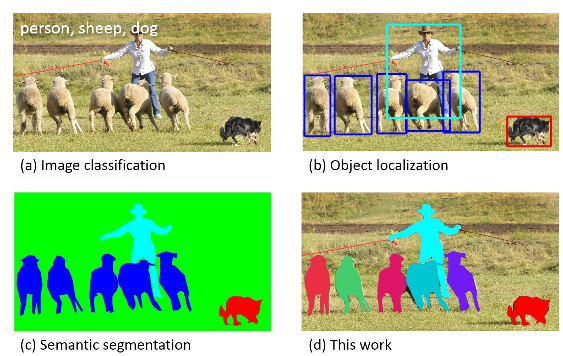Original article was published by Vijay Dubey on Deep Learning on Medium• Terminology
• References

## Terminology

• Classification(a): Just predicting the class of the objects present in the image.
• Object Detection(b): Classification as well as localization of the objects by predicting bounding boxes
• Semantic Segmentation(c): Partition the image into semantically meaningful parts, and to classify each part into one of the pre-determined classes, in othere words all pixels classified as belonging to one particular class are grouped together.
• Instance Segmentation(d): Segment out each instance present in the image separately, irrespective of them belonging to the same class or not.

## Novelty

• Mask RCNN focusses on pixel-to-pixel alignment,which is the main missing piece of Fast/Faster R-CNN, by replacing ROIpool with ROIalign layer and this achieves improvement in predicting not only the masks, but also improves the classification and detection predictions significantly.
• Also, mask and class prediction are decoupled, unlike the FCNs.A binary mask is predicted for each class independently, and the network’s RoI classification branch predicts the category. In contrast, FCNs usually perform per-pixel multi-class categorization,which couples segmentation and classification, and works poorly for instance segmentation

We will discuss both of these ahead.

## Architecture

• Two models have been experimented for the backbone(similar to the Faster R-CNN)architecture for feature extraction, namely ResNets and FPN(Feature Pyramid Networks)
• MaskRCNN adds a third branch(in parallel), that outputs the object mask, with the two output branches of Faster R-CNN(discussed above) for each candidate object.

We observe from the above image that the mask branch outputs `K mxm binary masks` for each RoI, where K is the number of classes(80 here, for COCO). This follows that each layer in the mask branch must maintain the explicit m×m object spatial layout, which in turn requires that the feature maps be well aligned with the image to preserve the explicit per-pixel spatial correspondence, which is ensured by the RoIalign layer, as discussed below.

## ROI Align

RoI pooling contains two step of coordinates quantization: from original image into feature map (divide by stride) and from feature map into roi feature (use grid). For example in ROIpool, for the feature map, we calculate [x/16] for each x on the image, where 16 is a feature map stride and likewise, quantization is performed when dividing into bins like 2X2 above.Those quantizations cause a huge loss of location precision. In ROIalign, we use x/16 to properly align the extracted features with input pixels. See the example below:

To select 15 pixels from the original image, we just select 15 * 25/128 ~= 2.93 pixels while In RoIPool, we would have rounded and selected 2 pixels causing a slight misalignment. Also, we use bilinear interpolation to get a precise idea of what would be at pixel 2.93.

## Bilinear Interpolation

We spent quite a lot of time understanding this and how is it used to estimate the pixel values at non-integer points. Here is a brief overview of the approach:

## RoIPool vs RoIAlign

Doing RoIPool, if the expected warped output size is 2×2, we quantize the division, by taking floor(x/2) ,ceil(x/2) and floor(y/2) ,ceil(y/2), where x and y are width and height of the RoI. Then maxpooling is done in each of the bins to get a 2×2 size output, as shown below:

For RoIAlign, as described in the paper, firstly, the RoI is divided into equal dimension bins(3.5×2.5 in this case) and then, we use bilinear interpolation to compute the exact values of the input features at four regularly sampled locations(indicated by red x) in each RoI bin, and aggregate the result(using max or average).

## Loss Function

The multi-task loss function is a sum of classification, localization and segmentation loss, defined as :

The former two are same as Faster RCNN. Since, for the mask branch, the total output is of size Km². To this a per-pixel sigmoid is applied, and Lmask is the average binary cross-entropy loss. Note that, not all the K masks contribute to the loss, but only the p-th mask, where p is the class label of the ground truth box, to which that RoI is associated.

Note: The mask target is the intersection between an RoI and its associated ground-truth mask